# Kidspiration Templates

Kidspiration Templates and Tutorials
 Fractional Parts: Students click on the object or section of an object to "color" and then click on the "symbol colors" icon on the bottom yellow bar. A color palette will appear and students choose the desired color from the palette. Denominators of the fractional parts are 2, 3 and 4 in this template which is designed for grade 3. The template includes a link to an interactive math site for more practice with fractions.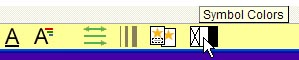Fractional Parts: The demoninators of the fractional parts in this template are 2,3,4,6 and 10. It is designed for grade 4. Students fill in the fractional parts by using the color palette as described for the template above. There are hyperlinks to interactive websites for more practice with fractions - "Visualizing Fractions" and "Equivalent Fractions."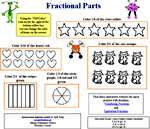Multiplication: This template on multiplication is divided into tree parts: Making 3 different arrays with 24 boxes Equally distributing 48 marbles into 6 bags Hyperlink to an interactive website where students can practice multiplication facts - "Ambelweb Tables Tester." Students are also expected to type the number sentence to match the objects on the screen.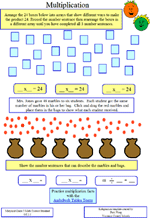Who's Tallest?: Students are sent on a mission with this Kidspiration template. They are given the heights of 4 students in 4 different measurements and are asked to arrange the students in order from shortest to tallest. A hyperlink to a conversion website is provided as well as some strategies to help them organize their thoughts and plans for solving the challenge. The template includes a second hyperlink which takes the students to an interactive website where they can practice identifying whole numbers and decimals on a number line. The number line activity is best used as a teacher directed activity. Create a number line (using a large screen display) and cover two of the numbers. See if the students can identify the covered numbers. Ask the students if they can create a number line where the third number in the pattern is 8. (2, 4, 8) Following this pattern, what would be the 4th or 5th number?Place Value: In this template students review their knowledge of place value in several ways: For given numbers they identify the number in a specific place value They arrange numbers in order from least to greatest. They write numbers in expanded form. They place a number on the correct place on a number line The template has a hyperlink to a website where students can have more practice with place value.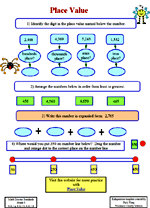Angles and Line Segments: Students will have fun creating angles with the "symbol maker" in Kidspiration. After they create their angles and lines they must place them in the correct box on the screen. The hyperlink in the template takes students to an online geoboard where they can make other shapes and angles according to the direction of their teacher.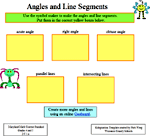Factor Trees: Students create factor trees for the numbers 30, 28 and 42 using the sample shown on the template. A link to an interactive math website takes the students to a webpage where they can practice more factoring.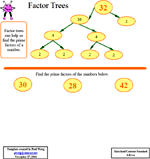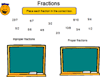ordering fractions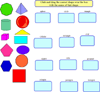matching shapes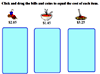money up to \$3ordering numbers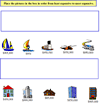ordering numbers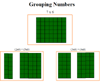equations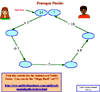facts puzzle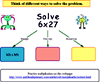equations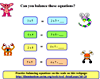balancing equations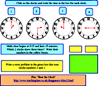telling time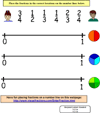comparing fractions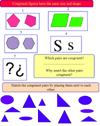congruent shapesfacts puzzlesadding money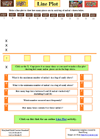line plot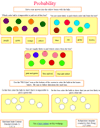probabilityFractional parts of a group (gr. 3)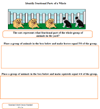Fractional parts of a group (gr. 4)